## Thursday, December 29, 2011

### Malware Analysis Tutorial 9: Encoded Export Table

Learning Goals:

1. Practice reverse engineering techniques.
2. Understand basic checksum functions.
Applicable to:
1. Operating Systems.
2. Computer Security.
3. Assembly Language

1. Introduction
This tutorial answers the challenges in Tutorial 8. We explain the operations performed by Max++ which are related to export table. In this tutorial, we will practice analyzing functions that do not follow C language function parameter conventions, and examine checksum and encoding functions.

2. Lab Configuration
You can either continue from Tutorial 8, or follow the instructions below to set up the lab. Refer to Tutorial 1 and Tutorial 4 for setting up VBOX instances and WinDbg.
(1) In code pane, right click and go to expression "0x40105c"
(2) right click and then "breakpoints -> hardware, on execution"
(3) Press F9 to run to 0x40105c
(4) If you see a lot of DB instructions, select them and right click -> "During next analysis treat them as Command".
(5) Exit from IMM and restart it again and run to 0x40105c. Select the instructions (about 1 screen) below 0x40105c, right click -> Analysis-> Analyze Code. You should be able to see all loops now identified by IMM.

3. Analysis of Code from 0x40108C to0x4010C4
We now continue the analysis of Tutorial 8, which stops at 0x401087.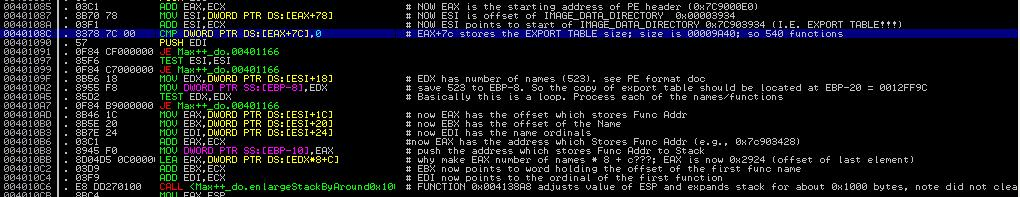Figure 1. Code from 0x40108C to 0x4010C4
Recall that EAX at this moment points to the beginning of DLL base. From Figure 1 of Tutorial 8, we can infer that offset 120 (0x78), it is the EXPORT TABLE beginning address, and at offset 124 (0x7c), it is the EXPORT TABLE size. So the instruction COMP DWORD DS:[EAX+7C], 0 is to compare the size of export table with 0. Clearly, the JE instruction at 0x401091 will not be executed and the control flow will continue to 0x40109F.

Now let's observe the instruction MOV EDX, DWORD PTR DS:[ESI+18].  Note that ESI, after being set by instruction at 0x40108A, is now pointing at the export table. It contains value 0X7C903400 (which is the starting address of the export table). In Section 3 of Tutorial 8, we have given the data structure of the export table. From it, we can infer that offset 0x18 is the number of names. Thus, after the instruction is executed, EDX has the number of names (0x523) exported from ntdll.dll.

Using the same technique, we can infer that the subsequent instructions (0x4010AD to 0x4010C4) assigns registers EAX to EDI. We have:

EAX <-- offset (relative to 0x7C90000 starting of ntdll base) of the beginning address of the array that stores function entry addrsses
EBX <-- offset of the beginning of the array that stores the names of the functions
EDI <-- offset of the array that stores the name ordinals (as we mentioned earlier, to find the entry address of a function, we have to find its index of the function name in the function name array, and then use the index to find the ordinal, and then use the ordinal to locate the entry address in the array of function entry address)

We can verify the above analysis. Take EBX as one example, its value is 0x7C9048B4. The following is the dump of the memory starting from that address. Note that each element is the "offset of the name". So the first element is 0x00006790 (it actually means that the string is located at 0x7C906790), and similarly, the second string is located at 0x7C9067A9. Figure 3 now displays the contents at 0x7C906790 (you can see that the first function name is CsrAllocateCaptureBuffer).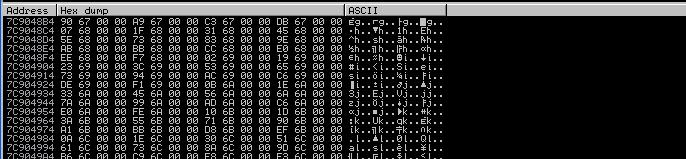Figure 2: Array of Function Names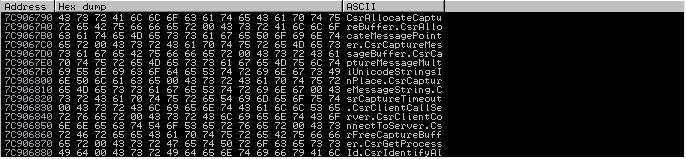Figure 3: The Strings

4. Analysis of Function 0x004138A8
Now we proceed to the instruction at 0x004010C6 (see Figure 1). It calls the function located at 0x004138A8. Figure 4 shows a part of the function.Figure 4: Function 0x004138A8
Notice that malware authors will not simply follow C language calling conventions (i.e., to push parameters into stack). Instead, they may use registers directly to pass information between function calls. To analyze the functionality/purpose of a function, we need to figure out: (1) what are the inputs and outputs? (2) and then the logic of the function.

To figure out the input parameters of the function, we look at those registers that are READ before assigned. Looking at the instructions beginning at 0x004138A8, we soon identify that EAX is the input parameter, at this moment its value is 0x00002924. Recall that in Section 3, it contains the offset of the beginning of the array that contains function entry addresses, i.e., 0x7C902924 is the beginning of the array that contains function entry addresses.

Then starting from 0x004138A9, the next few arithmetic instructions seem to be rounding the value of ECX based on EAX. Now Figure 5 shows the second half of the function. There are two interesting instructions, first the instruction at 0x0041389A (it is to reduce the value of EAX by 0x1000 inside a loop), and then the instruction of 0x413893, it is to exchange the value of EAX and ESP.

So the eventual output is the ESP register, which has multiples of 0x1000 bytes (in our case 0x2000 bytes) reduced compared with its original value.

In a word, the function at 0x004138A8 expands the stack frame (recall the stack grows from higher address to lower address) by 0x2000 bytes. Why? It is used to hold the new export table, which is encoded!

Challenge 1 of the Day: where does the new export table start?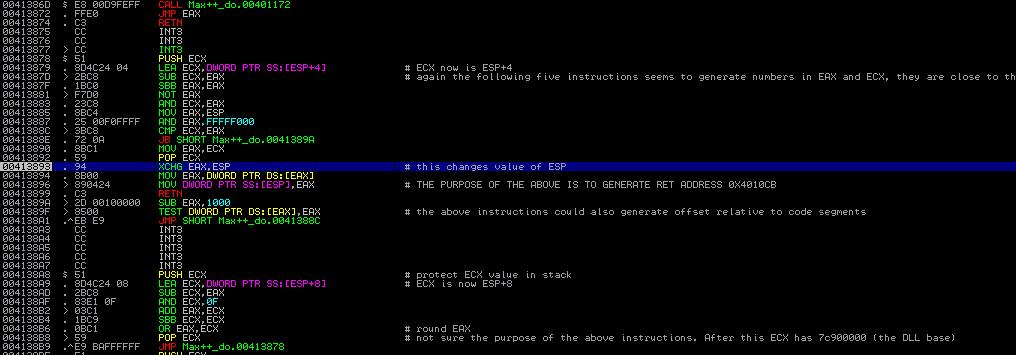Figure 5: Second Half of Function 0x004138A8
5. Rest of Encoding Function
We now proceed to analyze the code between 0x4010CB to 0x40113B. Note that the expanded stack now includes around 0x2000 bytes from 0x0012D66C. This is going to hold encoded export table. This encoded table (its format) is defined by the malware author himself/herself. Each entry has two elements and each element 4 bytes. The first element is the checksum of the function name, and the second is the function address. Later, the Max++ malware is able to invoke the system functions in ntdll.dll without resolving them using the export table of ntdll.dll, but using its own encoded table.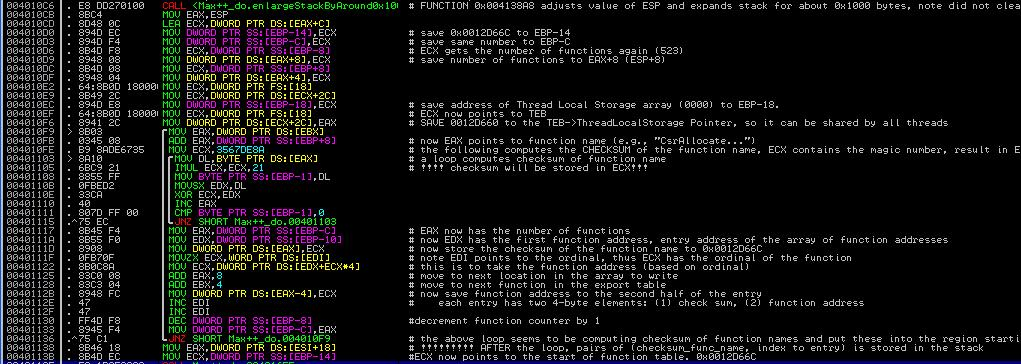Figure 6. Code from 0x4010CB to 0x40113B
Most of the program logic is pretty clear. Instructions from 0x4010D0 to 0x40DF save some important information to stack. Now [EBP-C] and [EBP-14] both have value 0x0012D66C (which is ESP+C). This is going to be the beginning of the encoded table, which will be demonstrated by the code later.

Then there is a 2-layer nested loop from 0x004010F9 to 0x00401136. Let's first look at the inner loop from 0x401103 to 0x401110. Clearly, EAX is the input for this inner loop and note that EAX is pointing to the array of chars that represent the function name (at 0x004010FB). At 0x401110, EAX is incremented by one in each iteration of the inner loop. Clearly, the inner loop is doing some checksum computation of the function name. In the checksum loop, there are two registers being written by the code: EDX and ECX. If you read the code carefully, you will note that the value of EDX is overwritten completely in each iteration (note instruction at 0x401103). Only ECX's previous value affects its next value. Thus ECX must be the output and it saves the checksum!

Then the instructions from 0x401117 to 0x401133 are to set up the entry for the function. The instruction at 0x40111D (MOV DS:[EAX], ECX) is to save the checksum of the function to the first element, and then the instruction at 0x0040112B saves the function address as the second element.

Challenge 2 of the Day: Explain the logic of EDX+ECX*4 of the instruction at 0x00401122. Hint: study the use of ordinal numbers in export table.

5. Conclusion
Our conclusion is: Max++ reads the export table of ntdll.dll and builds an encoded export table for itself. We will later see its use.

6. Challenge of the Day
At 0x0040113E,  Max++ calls 0x0040165E. Analyze the functionality of 0x0040165E.

1.thanks bro Dr. Fu's , good article.:-*:x

2.Very interesting behavior from Max++.

3.Ordinal value is actually the index of the AddressOfFunctions,The array is build from DWORD elements, This means that the value should be multiplied by four to get the RVA of a function.

Dana Feigel

4.Dr.Fu, Excellent tutorials. Truly enjoyable.

Had 1 doubt . What is the significance of 7C902924? You have said that it points to beginning address of the array that stores function entry addrsses. Isn't 7C903428 pointing to that?

Thanks,
Jutsin

5.Your tutorial very good you solve many thing in codec thanks for share it engineering statement of purpose .

6.Very well written and easy to understand. Have taken our relevant point out of this blog and will surely pass it onto our configuration management team to put in a tool which does this metrics. Will be really helpful.

Source code security analysis

7.thank you so much for this good informations !

ก็อตซิลล่า 2015

8.9.10.Thanks for sharing, very informative blog.
ReverseEngineering

11.Nice blog!!!!!!!.
ReverseEngineering

12.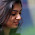Thanks for your informative article, Your post helped me to understand the future and career prospects & Keep on updating your blog with such awesome article.
Docker online training
Docker certification training
Docker online course
Docker training course

13.This is most informative and also this post most user friendly and super navigation to all posts. Thank you so much for giving this information to me.Docker training in Chennai.
Java training in chennai | Java training in annanagar | Java training in omr | Java training in porur | Java training in tambaram | Java training in velachery